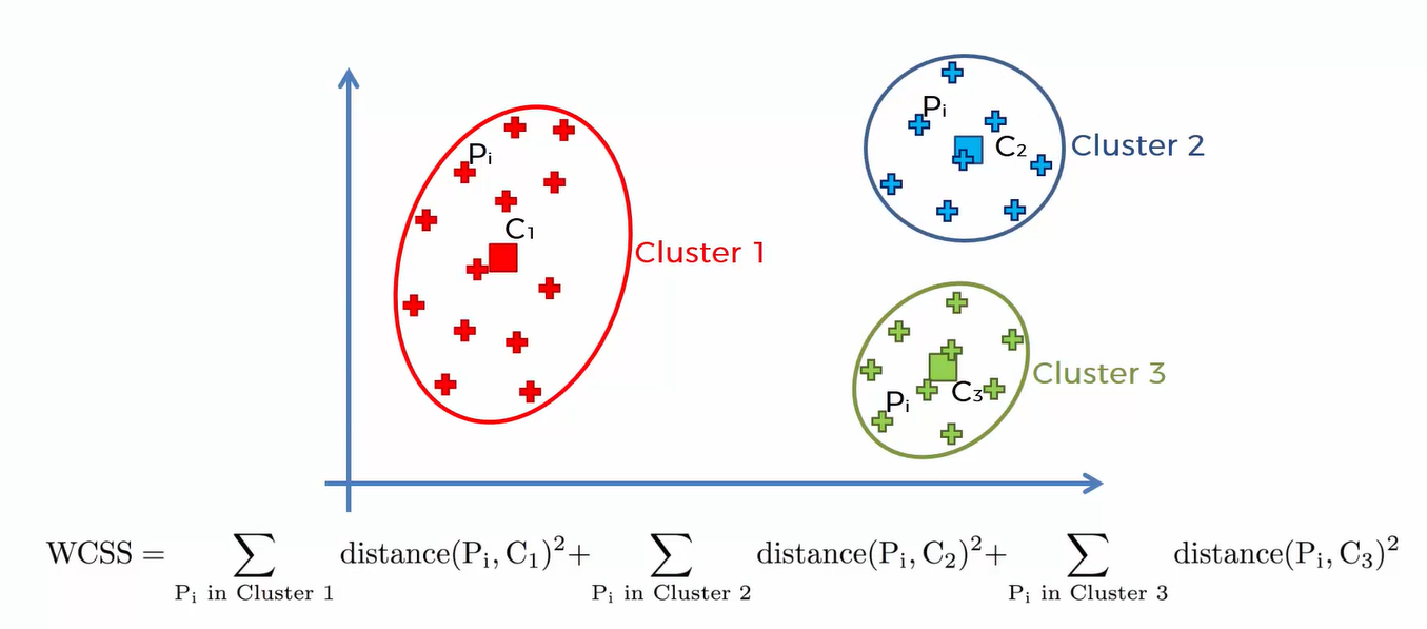# How to choose the Right Number of Clusters in the K-Means Algorithm?

Original article was published by Manik Soni on Deep Learning on Medium# How to choose the Right Number of Clusters in the K-Means Algorithm?

## What is Within-Cluster-Sum-of-Squares(WCSS) in clustering? The Elbow method used in K-Means Algorithm.

Before we dive deep into choosing the right number of clusters in the K-Means Algorithm, we first Know What is K-Means Algorithm?

Now, the important question is:

# How to choose the Right Number of Clusters in the K-Means Algorithm?

So in order to choose the right number of clusters, we first take an example of this ‘scatter’ plot :

So, our result looks like this,

But, how we can able to take 3 clusters for doing categorization? why can’t 2 or 4?

To answer this, Let’s understand the concept stepwise:

Step 1. First, we understand What is Within-Cluster-Sum-of-Squares (WCSS)?

WCSS may be defined as an Implicit Objective Function which helps to give the right number of centroids or clusters to include in the dataset.

It gives the measure of the sum of distances of observations from their cluster centroids.

Step 2. Now let’s include 1 centroid in our dataset. Now the value of WCSS is very high because if we do the calculation the sum of distances of observations from their cluster centroids gives a very big result.

Step 3. Now include one more centroid that is, include 2 centroids in the dataset.WCSS result is much less as compared to the Step 2 result.

Step 4. Now again include one more centroid that is 3 centroids in the dataset. It gives a much lower result of WCSS than Step 3.

Step 5. Now, the question is when to stop adding the centroids into the dataset?

In order to answer this, let’s analyze the sequential steps of adding the centroid.

So, According to the above graph, we can analyze the substantial change in the value of WCSS by adding 2 centroids from 1 centroid.

Again, see the abrupt change by adding 3 centroids from 2 centroids.

By adding centroids from 3 to 10, you can see that there is no abrupt change but a small difference observed while adding new centroids.

So centroid 3 is a threshold that gives us a value of how much clusters to include in our dataset?

This Method of finding is known as the Elbow method.